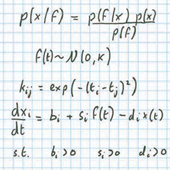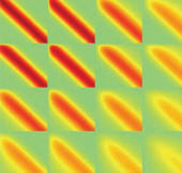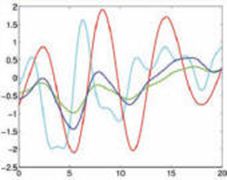# Computational Biology in Neuroscience

##It is over 300 years since Isaac Newton described a set of mathematical rules that predicted the motion of the planets. These mathematical rules held with such generality that not only could they be used to predict the flight of projectiles in war, but they also allowed Carl Friedrich Gauss to predict the orbit of the dwarf planet Ceres, allowing its rediscovery 11 months after it was lost behind the Sun. Gauss used the physical principles encoded in Newton’s maths and determined the parameters of the system (e.g. the mass of the planet) using least squares fits to the observations of the orbit. The same principles were also used to predict the flight of spacecraft and plan our path to the moon.

##### Mathematical modelling in translational medicine

There is a thread that joins the fundamental work of Newton and Gauss to mathematical modelling in translational medicine. The key idea is to use a mathematical abstraction, or model, of the system. The parameters of the model are then inferred through observing data from the system. In our research the systems of interest are not planets, but human cells. The mathematical models we use are far more complex than those wielded by Gauss, but yet they are still crude when compared to the complexity of the cells we hope to understand. The data are far more numerous than the observations of Ceres’s orbit, but yet they are often still too scarce to make confident predictions with the model. These issues leave us with uncertainty. Uncertainty about the quality of the model we use; uncertainty about the parameters we infer.

##### From uncertainty to confidence: The Bayesian inference model

Fortunately a methodology exists to make confident predictions in the light of all these problems. The methodology was developed mainly by a contemporary of Gauss: Pierre Simon Laplace. His initial interest was whether or not a coin was biased. For Laplace’s coin the parameter of interest is the probability of a heads, and the observations consist of the results of coin flips. As you increase the number of coin flips you become more confident about whether the coin is biased. Laplace introduced an approach now known as Bayesian inference (after Thomas Bayes who independently discovered the same principle) for quantifying the uncertainty associated with parameters. Bayesian inference makes clear the importance of doing the right experiment and making the right measurement. This allows us to find better models and become more confident about our predictions.

##### Modelling disease to predict outcomesModern translational modelling is a synthesis of the ideas of Newton, Gauss and Laplace. The mathematics acts as a toolkit to improve our understanding of the system. This then allows us to make confident predictions about the nature of a response to a given treatment: just as NASA was able to make confident predictions about the fuel required to land a lunar orbiter. At SITraN we do this by working closely with clinicians and neuroscientists. The mathematical model assimilates data from clinical studies and biological experiments. As we acquire more data we can improve the model and help in the design of the next experiment. Through iterating between mathematical models and experiments we can improve our understanding of disease and deliver more effective treatments.